almost 9 years ago

## 機器學習 -- Logistic Regression Model

### 1.Introduction

Logistic Regression是一種機器學習的Model,可以用來處理分類問題

Logistic Regression的model如下

$X$是input data, $W$ 是weight,這兩者皆是矩陣

$\eta$ 是學習速度,可以調整演算法收斂的速度

### 2. Draw 3D Logistic Model

Z=np.divide(1,1+np.exp(np.multiply(w0,X0)+np.multiply(w1,X1)+np.multiply(w2,X2)))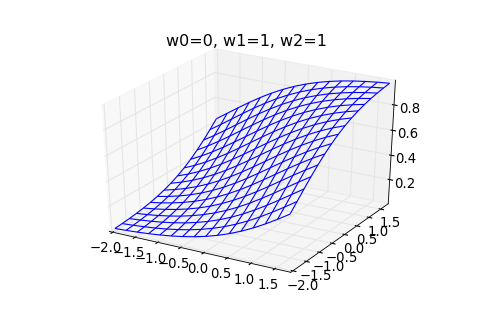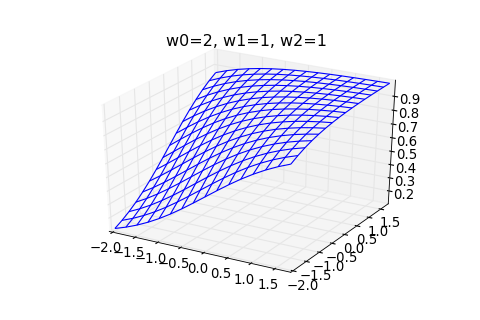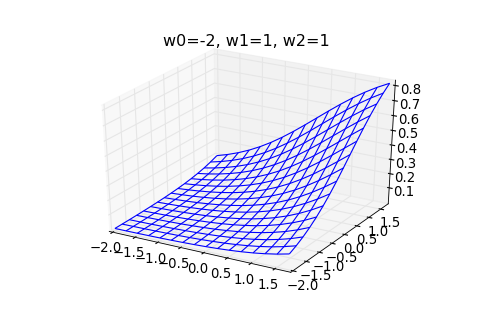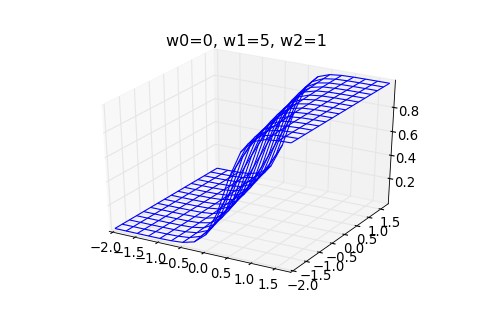### 3. Learning by Logistic Model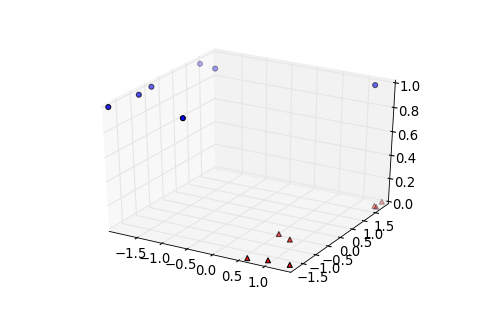logistic_model.py 加入一個functionlogistic_model.py 加入以下function

times是這個迴圈要跑幾次,
eta是學習速率,如果eta越小,就越慢收斂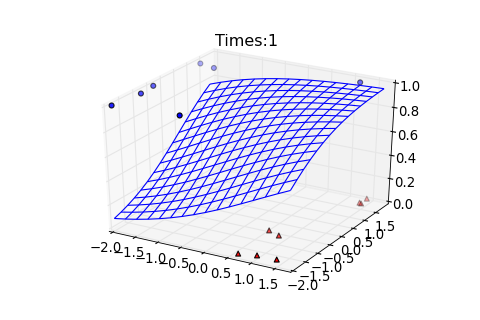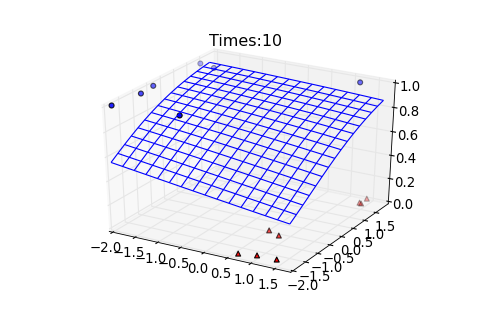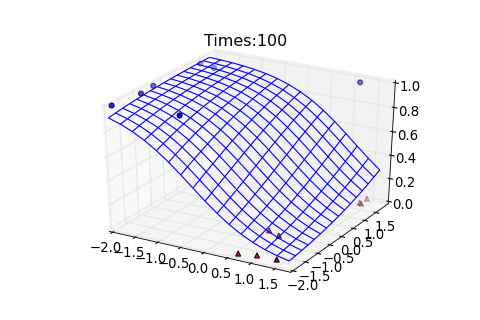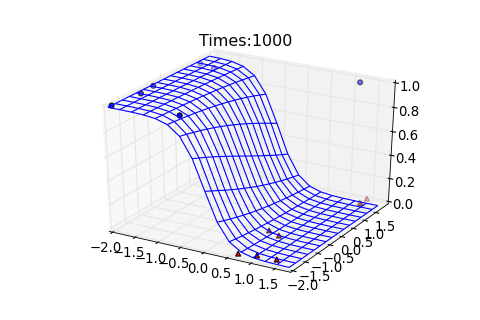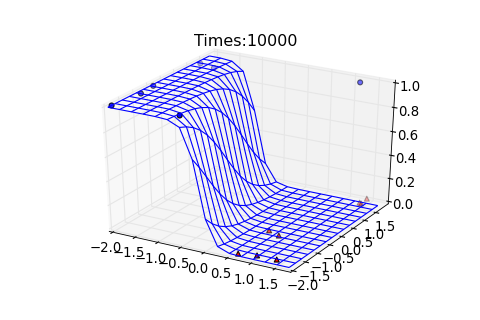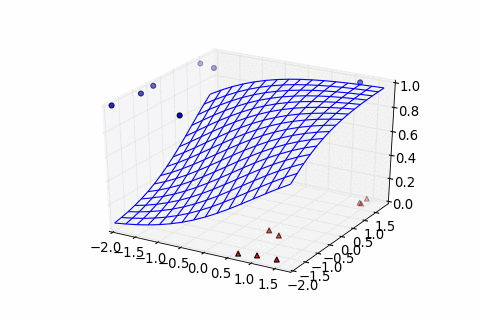model可以符合大部分的data都可以達到準確預測

##### 1.Andrew Ng. Machine Learning

Andrew Y. Ng 是Stanford University的知名教授,也是Google Brain開發團隊的成員之一

https://www.coursera.org/course/ml

##### 2.林軒田 機器學習基石 (Machine Learning Foundations)

https://www.coursera.org/course/ntumlone